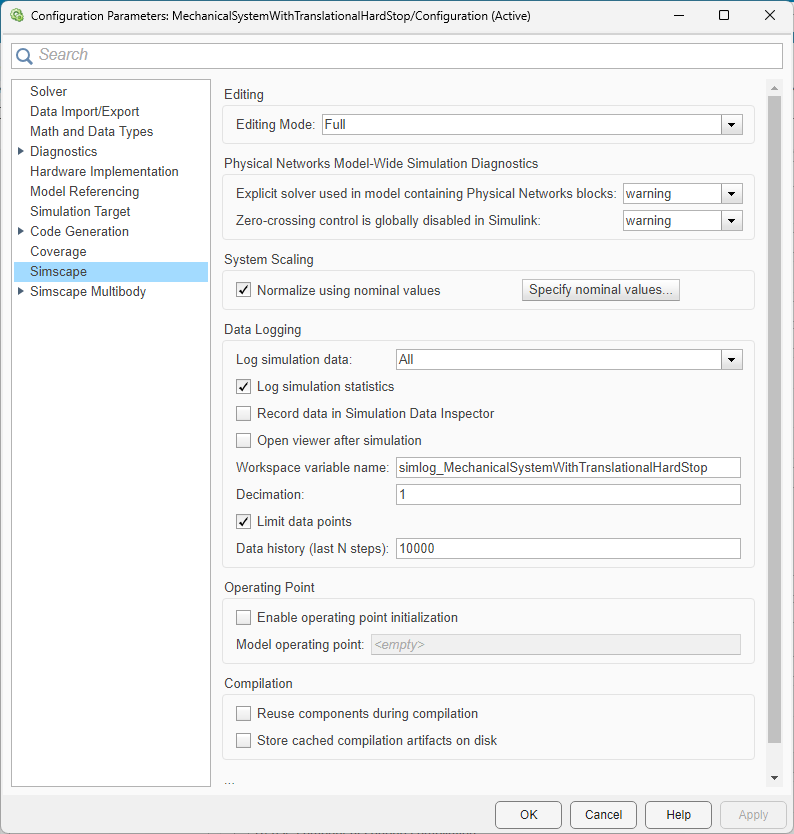## Log Simulation Statistics

This example shows how you can access and analyze information on zero crossings during simulation. By default, the zero-crossing data is not logged. If you select the Log simulation statistics check box, the simulation log variable contains an additional `SimulationStatistics` node for each block that can produce zero crossings, at the price of slower simulation speed and heavier memory consumption.

1. Open the Mechanical System with Translational Hard Stop example model by typing `ssc_mechanical_system_translational_hardstop` in the MATLAB® Command Window.2. Open the Configuration Parameters dialog box and then, in the left pane, select Simscape. You can see that this example model already has data logging for the whole model enabled, as well as simulation statistics, and that the workspace variable name is `simlog_ssc_mechanical_system_translational_hardstop`.3. Simulate the model. This creates a workspace variable named `simlog_ssc_mechanical_system_translational_hardstop` (as specified by the Workspace variable name parameter), which contains the simulation data. Because you selected the Log simulation statistics check box, the workspace variable contains additional nodes that represent zero-crossing data.

4. The `simlog` variable has the same hierarchy as the model. To see the whole variable structure, at the command prompt, type:

`simlog_ssc_mechanical_system_translational_hardstop.print`

This command prints the whole data tree.

``` ssc_mechanical_system_translational_hardstop +-Damper_M1 | +-C | | +-v | +-R | | +-v | +-f | +-v +-Damper_M2 | +-C | | +-v | +-R | | +-v | +-f | +-v +-MTRef_DM1 | +-V | +-v +-MTRef_DM2 | +-V | +-v +-MTRef_VS | +-V | +-v +-Mass_1 | +-M | | +-v | +-f | +-v +-Mass_2 | +-M | | +-v | +-f | +-v +-Sensor_M1 | +-Ideal_Translational_Motion_Sensor | | +-C | | | +-v | | +-P | | +-R | | | +-v | | +-V | | +-f | | +-v | | +-x | +-MTRef | | +-V | | +-v | +-PS_Terminator | | +-I | +-PS_Terminator1 | +-I +-Sensor_M2 | +-Ideal_Translational_Motion_Sensor | | +-C | | | +-v | | +-P | | +-R | | | +-v | | +-V | | +-f | | +-v | | +-x | +-MTRef | +-V | +-v +-Spring_M1 | +-C | | +-v | +-R | | +-v | +-f | +-v | +-x +-Translational_Hard_Stop | +-C | | +-v | +-R | | +-v | +-SimulationStatistics | | +-zc_1 | | | +-crossings | | | +-values | | +-zc_2 | | +-crossings | | +-values | +-f | +-v | +-x +-Velocity_Source +-C | +-v +-R | +-v +-S +-f +-v```
5. Under the `Translational_Hard_Stop` node, there is a node called `SimulationStatistics`, which contains zero-crossing information. This means that Translational Hard Stop is the only block in the model that can generate zero-crossings during simulation.

6. You can access and analyze this data similar to other data that is logged to workspace during simulation. For more information, see `simscape.logging.Node` and `simscape.logging.Series` reference pages.